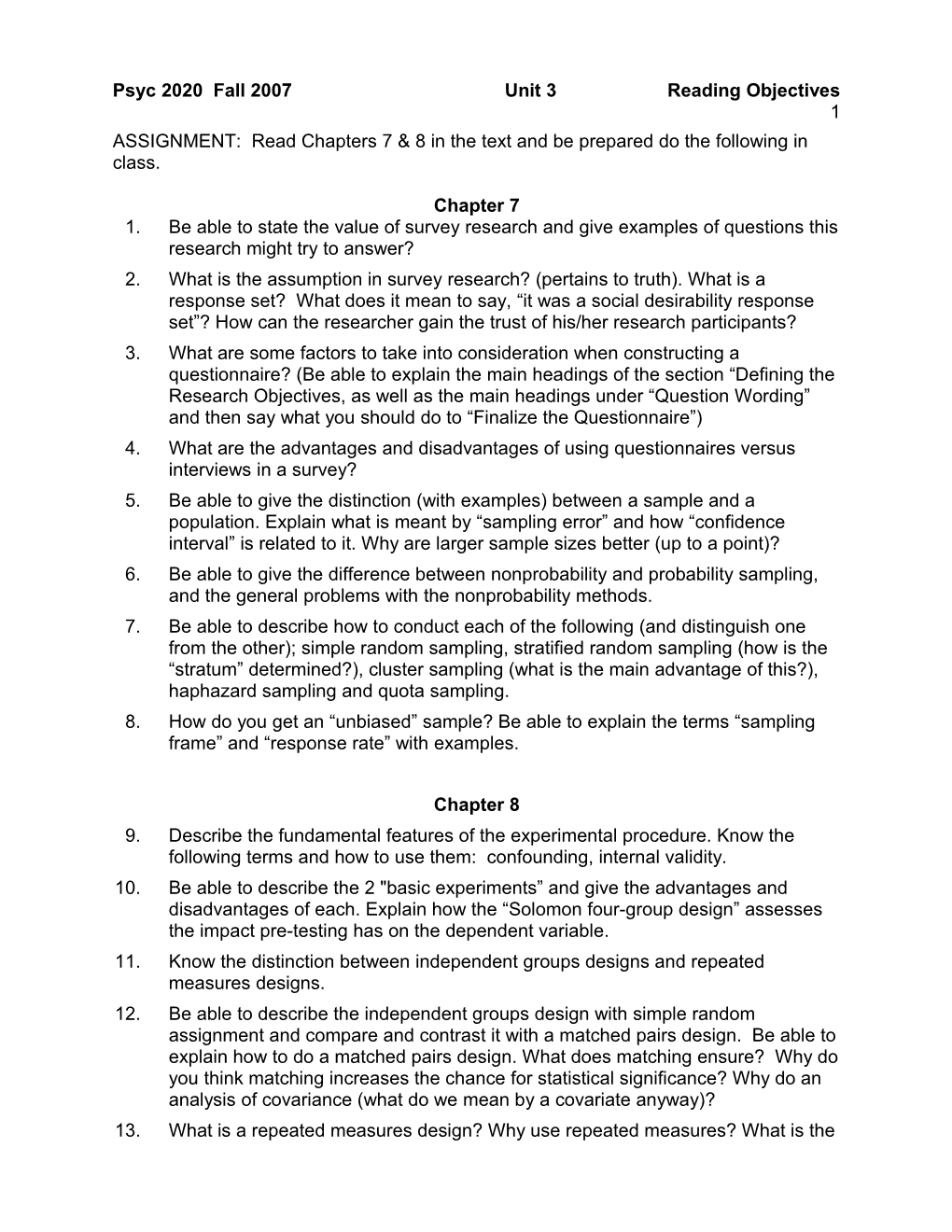# ASSIGNMENT: Read Chapters 7 & 8 in the Text and Be Prepared for an Exam Over the FollowingPsyc 2020 Fall 2007Unit 3Reading Objectives

1

ASSIGNMENT: Read Chapters 7 & 8 in the text and be prepared do the following in class.

## Chapter 7

1. Be able to state the value of survey research and give examples of questions this research might try to answer?
2. What is the assumption in survey research? (pertains to truth). What is a response set? What does it mean to say, “it was a social desirability response set”? How can the researcher gain the trust of his/her research participants?
3. What are some factors to take into consideration when constructing a questionnaire? (Be able to explain the main headings of the section “Defining the Research Objectives, as well as the main headings under “Question Wording” and then say what you should do to “Finalize the Questionnaire”)
4. What are the advantages and disadvantages of using questionnaires versus interviews in a survey?
5. Be able to give the distinction (with examples) between a sample and a population. Explain what is meant by “sampling error” and how “confidence interval” is related to it. Why are larger sample sizes better (up to a point)?
6. Be able to give the difference between nonprobability and probability sampling, and the general problems with the nonprobability methods.
7. Be able to describe how to conduct each of the following (and distinguish one from the other); simple random sampling, stratified random sampling (how is the “stratum” determined?), cluster sampling (what is the main advantage of this?), haphazard sampling and quota sampling.
8. How do you get an “unbiased” sample? Be able to explain the terms “sampling frame” and “response rate” with examples.

## Chapter 8

1. Describe the fundamental features of the experimental procedure. Know the following terms and how to use them: confounding, internal validity.
2. Be able to describe the 2 "basic experiments” and give the advantages and disadvantages of each. Explain how the “Solomon four-group design” assesses the impact pre-testing has on the dependent variable.
3. Know the distinction between independent groups designs and repeated measures designs.
4. Be able to describe the independent groups design with simple random assignment and compare and contrast it with a matched pairs design. Be able to explain how to do a matched pairs design. What does matching ensure? Why do you think matching increases the chance for statistical significance? Why do an analysis of covariance (what do we mean by a covariate anyway)?
5. What is a repeated measures design? Why use repeated measures? What is the major problem with this design?
6. Be able to explain the problem of order effects (and describe the types).
7. Be able to describe and differentiate between complete counterbalancing and Latin squares. What is the purpose of these manipulations?
8. Why should we be concerned about the time interval between treatments?
9. What needs to be considered when choosing between independent groups designs and repeated measures designs?
10. Be able to say (in general) what developmental psychologists study. Describe the two research methods commonly used (cross-sectional and longitudinal) and say what the strengths and weaknesses are for each method.